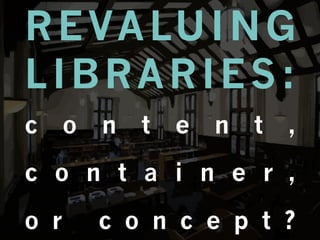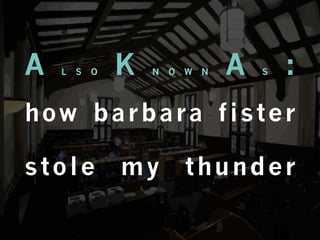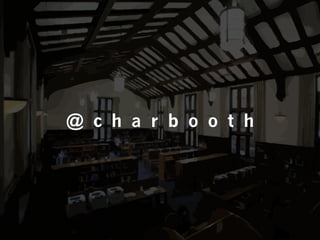Successfully reported this slideshow.

# Revaluing Libraries: Content, Container, or Concept?

12

Share×
1 of 145
1 of 145

# Revaluing Libraries: Content, Container, or Concept?

12

Share

Keynote given at the 2012 ACRL-Oregon conference in Corbett, Oregon, 26 October 2012. Audio available at http://bit.ly/acrlor12-boothaudio, video courtesy of Jim Holmes at http://www.youtube.com/watch?v=N16b2sMd_Ww.
.

Keynote given at the 2012 ACRL-Oregon conference in Corbett, Oregon, 26 October 2012. Audio available at http://bit.ly/acrlor12-boothaudio, video courtesy of Jim Holmes at http://www.youtube.com/watch?v=N16b2sMd_Ww.
.

## More Related Content

### Related Books

Free with a 14 day trial from Scribd

See all

### Related Audiobooks

Free with a 14 day trial from Scribd

See all

### Revaluing Libraries: Content, Container, or Concept?

1. R E VA LU I N G LIBRARIES: c o n t e n t , c o n t a i n e r , o r c o n c e p t ?
2. A L S O K N O W N A S : h ow b a r b a ra f i s t e r stole my thunder
3. @ c h a r b o o t h
4. N A R R AT I V E S
5. N A R R AT I V E S
6. 3 2 1
7. 3 2 1 DESTRUCTION
8. 3 2 DECLINE 1 DESTRUCTION
9. 3DISPLACEMENT 2 DECLINE 1 DESTRUCTION
10. 1 DESTRUCTION
11. 1 DESTRUCTION
12. 1 DESTRUCTION
13. 2 DECLINE
14. 2 DECLINE
15. 2 DECLINE
16. 3DISPLACEMENT
17. 3DISPLACEMENT 2 DECLINE
18. C O N T E N T
19. C O N T E N T C O N T A I N E R
20. C O N T E N T C O N T A I N E R C O N C E P T
21. reed
22. ✓ C O M I S S E R AT I O N
23. ✓ C O M I S S E R AT I O N ✓ GRAFFITING
24. ✓ C O M I S S E R AT I O N ✓ GRAFFITING ✓ MAKING OUT
25. ✓ C O M I S S E R AT I O N ✓ GRAFFITING ✓ MAKING OUT ✓ SLEEPING
26. OWNERSHIP
27. R I T U A L
28. COMMUNITY
29. E N G AG E M E N T
30. I N T E N T I O N E N G AG E M E N T
31. I N T E N T I O N D I S C I P L I N E E N G AG E M E N T
32. I N T E N T I O N L E A R N I N G D I S C I P L I N E E N G AG E M E N T
33. I N T E N T I O N L E A R N I N G E X P E R I E N C E D I S C I P L I N E E N G AG E M E N T
34. I N T E N T I O N L E A R N I N G K N O W L E D G E E X P E R I E N C E D I S C I P L I N E E N G AG E M E N T
35. C U L T U R E
36. M E M O R Y
37. M E M O R Y
38. C O N T E N T C O N T A I N E R
39. C O N T E N T C O N T A I N E R
40. C O N T E N T C O N T A I N E R
41. C O N T E N T C O N T A I N E R C O N C E P T
42. a c c e s s
43. a c c e s s freedom
44. a c c e s s freedom advocacy
45. a c c e s s freedom advocacy i n q u i r y
46. a c c e s s freedom advocacy i n q u i r y openness
47. a c c e s s freedom advocacy i n q u i r y openness
48. C O N T E N T
49. C O N T E N T C O N T A I N E R
50. C O N T E N T C O N T A I N E R C O N C E P T
51. O P E N SCHOLARSHIP AS P E DAG O G Y
52. p a u l o f r e i r e
53. p a u l o f r e i r e
54. j o h n d e w e y
55. j a c k m e z i r o w
56. b e l l h o o k s
57. CONTENT
58. C O N TA I N E R
59. CONCEPT
60. CHAR BOOTH instruction services M A N A G E R & e - l e a r n i n g L I B R A R I A N c l a re m o n t c o l l e ge s L I B R A R Y c h a r b o o t h @ g m a i l i n f o m a t i o n a l . c o m @ c h a r b o o t h t i ny u r l . c o m / p e a b o d y p o s t e r s slideshare.net/charbooth
61. SUGGESTED READING: 1 of 2 bennett, s. (2009). libraries and learning: a history of paradigm c h a n g e. p o r t a l : l i b r a r i e s a n d t h e a c a d e m y 9 ( 2 ) , 1 8 1 - 1 9 7 . r e t r i e v e d 1 0 o c t o b e r 2 0 1 1 , f r o m p r o j e c t m u s e d a t a b a s e. knuth, r. (2006). burning books and leveling libraries: extremist v i o l e n c e a n d c u l t u r a l d e s t r u c t i o n . w e s t p o r t , c o n n : p r a e ge r. knuth, r. (2003). libricide: the regime-sponsored destruction of books and l i b r a r i e s i n t h e t w e n t i e t h c e n t u r y . w e s t p o r t , c o n n : p r a e ge r. troll, d. (2001). how and why are libraries changing? digital librar y federation website, retrieved 10 october 2011, f r o m h t t p : / / o l d . d i g l i b . o r g / u s e / w h i t e p a p e r. h t m .
62. SUGGESTED READING: 2 of 2 accardi, drabinski, & kumbier. 2010. critical library instruction. dewey, john. 1916. democracy and education. freire, paulo. 1970. pedagogy of the oppressed. hooks, bell. 1994. teaching to transgress: education as the practice of freedom. mezirow, jack. 2009. transformative learning in practice: insights from community, workplace, and education.
63. I M A G E C R E D I T S y o u r s t r u l y o p e n c l i p a r t . o r g h u f f i n g t o n p o s t w i k i m e d i a c o m m o n s . o r g a l l e g r a g o n z a l e z h i s t o r y o f i n f o r m a t i o n . c o m s i l i c o n a n g l e . c o m u s g . e d u w w w. f l i c k r. c o m / p h o t o s / b a r t k i n g e p h e s u s t o u r s . c o m